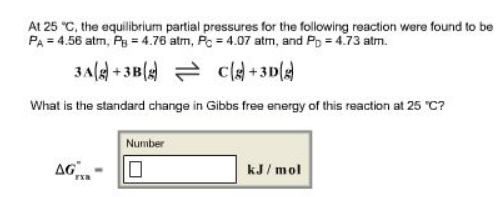# Problem: At 25°C, the equilibrium partial pressures for the following reaction were found to be PA = 4.56 atm, PB = 4.76 atm, PC = 4.07 atm, and PD = 4.73 atm. 3A(g) + 3B(g) ⇌ C(g) + 3D(g) What is the standard change in Gibbs free energy of this reaction at 25°C?

###### FREE Expert Solution
93% (413 ratings)###### Problem Details

At 25°C, the equilibrium partial pressures for the following reaction were found to be P= 4.56 atm, P= 4.76 atm, P= 4.07 atm, and P= 4.73 atm.

3A(g) + 3B(g) ⇌ C(g) + 3D(g)

What is the standard change in Gibbs free energy of this reaction at 25°C?